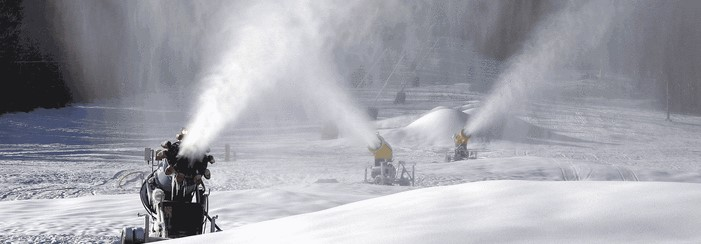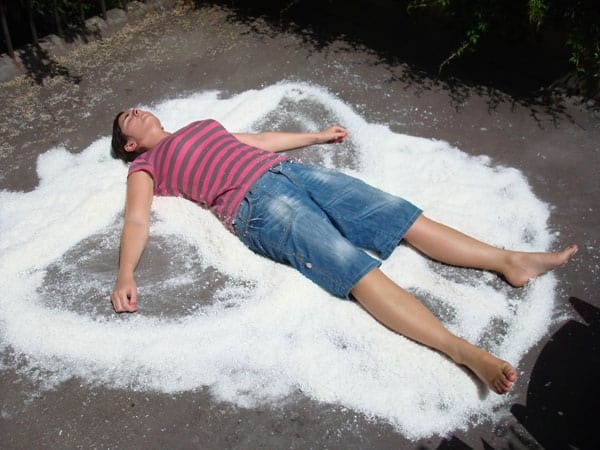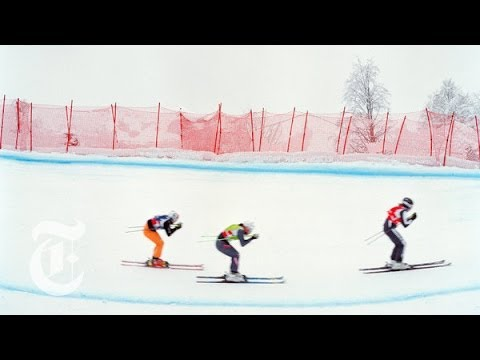# How To Make Fake Snow In SummerSkiing is a good idea to have a good time, especially, in the dead of winter, when a lot of people go to the ski resorts all over the world. But what to do, if the mother nature doesn’t provide us with enough amount of snow to have fun in summer? Or it actually doesn’t bring snow at all. Well, to make an artificial snow, we can use a snow-making equipment, and here we’re going to tell you how it works and disclose the secret of difference between the natural snow and the artificial one.

Okay, how does snow get formed in nature? High in the atmosphere, the tiny droplets of water freeze and create ice crystals. But the droplets of water can’t freeze even if the temperature is lower than -18 degrees Celsius. They need something that we used to call a “seed” – particles of dust for example. Then the droplets of water freeze around these seeds. Finally, the ice crystals collide with each other and create snowflakes.Machines that make snow like snow guns use the same principle as nature. To start the process of water droplets freezing, some tricks are used: compressed air and water are fed into the mixing chamber. The latter has the so-called “nucleating” nozzles.  In fact, the “nucleating” nozzles are fine holes in which compressed air and water are mixed. When air exits from the nozzles, it expands and cools rapidly that leads to  the water droplets freezing into tiny ice crystals.

Besides, the process in which the compressed air cools as it leaves the nozzles is called the Joule-Thomson effect. This effect defines the temperature change $dT$ of a gas forced through a porous plug between two vessels with the pressure $dP$:

$dT = \dfrac{1}{C_P}\left(T\left(\dfrac{\partial V}{\partial T}\right)_P – V\right)dP$

where $C_P$ is a heat capacity at a constant pressure. At this point, we should note that the right side of the equation above doesn’t equal zero only in case of a real gas, also known as Van der Waals gas. The state of a real gas can be introduced by its parameters $P, V, T$ as follows:

$\left(P+\dfrac{a}{V^2}\right)\left(V-b\right)=RT$

here $a$ and $b$ are the correction coefficients that account additional attraction forces between particles and their real sizes.

Now, to prove that physics knowledge can be useful in a daily life, let’s make an estimation of air temperature change during the Joule-Thomson process. For this, we need to calculate derivation $\left(\dfrac{\partial V}{\partial T}\right)_P$ from equation of gas state:

$\dfrac{\partial}{\partial T}\left[\left(P+\dfrac{a}{V^2}\right)\left(V-b\right)\right]_P = \dfrac{\partial}{\partial T}\left[RT\right]_P$

$\left(\dfrac{\partial V}{\partial T}\right)_P=\dfrac{R(V-b)}{RT-\dfrac{2a}{V^3}(V-b)^2} \approx \dfrac{V-b}{T\left(1-\dfrac{2a}{RTV}\right) }$

Molar volume of the Van der Waals gas can also be easily derived from the equation of gas state:

$V\approx \dfrac{RT}{P}-\dfrac{a}{RT}+b$

This allows us to construct full equation for the temperature change:

$dT = \dfrac{1}{C_P}\left(\dfrac{V-b}{1-\dfrac{2a}{RTV}}-V\right)dP$

Using linear approximation, we can roughly evaluate given expression in the following form:

$\int_{T_1}^{T_2}dT = (T_2-T_1) = \dfrac{1}{C_P}\left(\dfrac{V_1-b}{1-\dfrac{2a}{RT_1V_1}}-V_1\right)(P_2-P_1)$

Now suppose that in the first chamber, we have compressed air at room temperature ($T_1=293 K$) and pressure $P_1=6 atm = 6\times 10^5 Pa$. And we throttle it to the atmosphere (pressure $P_2 = 1 atm$). Correction coefficients for the air are well known, they are $a=1.3 \dfrac{Pa\cdot m^6}{mol^2}$ and $b=114\times 10^{-6}\dfrac{m^3}{mol}$. Then, according to the formula, $T_2-T_1=-23 K$.

Now, after all this physics stuff, enjoy the video and cool down your friends with some surprising facts about snowmaking.2 Shares
Filed under Physics.